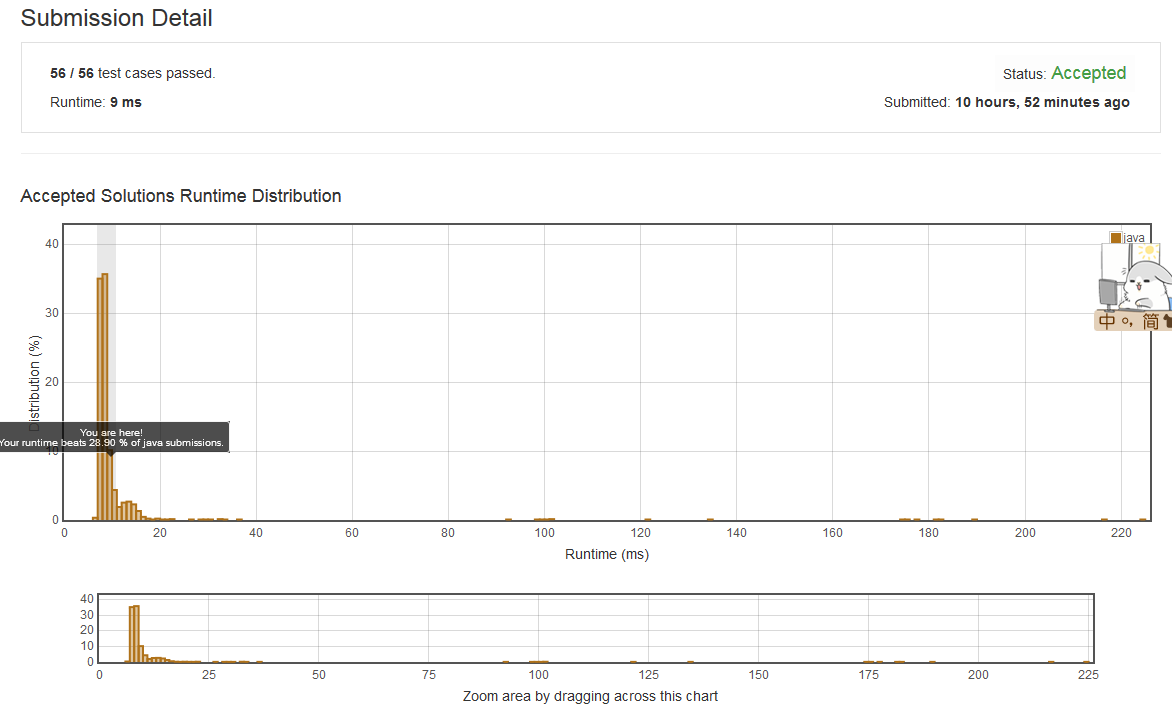# LeetCode 566 Reshape the Matrix

In MATLAB, there is a very useful function called 'reshape', which can reshape a matrix into a new one with different size but keep its original data.

You're given a matrix represented by a two-dimensional array, and two positive integers r and c representing the row number and column number of the wanted reshaped matrix, respectively.

The reshaped matrix need to be filled with all the elements of the original matrix in the same row-traversing order as they were.

If the 'reshape' operation with given parameters is possible and legal, output the new reshaped matrix; Otherwise, output the original matrix.

Example 1:

Input:
nums =
[[1,2],
[3,4]]
r = 1, c = 4
Output:
[[1,2,3,4]]
Explanation:
The row-traversing of nums is [1,2,3,4]. The new reshaped matrix is a 1 * 4 matrix, fill it row by row by using the previous list.




Example 2:

Input:
nums =
[[1,2],
[3,4]]
r = 2, c = 4
Output:
[[1,2],
[3,4]]
Explanation:
There is no way to reshape a 2 * 2 matrix to a 2 * 4 matrix. So output the original matrix.




Note:

1. The height and width of the given matrix is in range [1, 100].
2. The given r and c are all positive.

class Solution {
public int[][] matrixReshape(int[][] nums, int r, int c) {
int m=nums.length;
int n=nums.length;

if(r*c == m*n) {

int[] array = new int[m*n];
for(int i=0; i<m; i++) {
for(int j=0; j<n; j++) {
array[i*n+j] = nums[i][j];
}
}

int[][] newMatrix = new int[r][c];
for(int i=0; i<r; i++){
for(int j=0; j<c; j++) {
newMatrix[i][j] = array[i*c+j];
}
}

return newMatrix;
} else {
return nums;
}
}
}class Solution {
public int[][] matrixReshape(int[][] nums, int r, int c) {
int m=nums.length;
int n=nums.length;

if(r*c != m*n) {
return nums;
}

int[] array = new int[m*n];
for(int i=0; i<m; i++) {
for(int j=0; j<n; j++) {
array[i*n+j] = nums[i][j];
}
}

int[][] newMatrix = new int[r][c];
for(int i=0; i<r; i++){
for(int j=0; j<c; j++) {
newMatrix[i][j] = array[i*c+j];
}
}

/* int[][] newMatrix = new int[r][c];
for(int i=0; i<r; i++){
for(int j=0; j<c; j++) {
newMatrix[i][j] = nums[i]*c+j];
}
}
*/

return newMatrix;

}
}posted @ 2018-04-13 09:43  Zhao_Gang  阅读(91)  评论(0编辑  收藏  举报